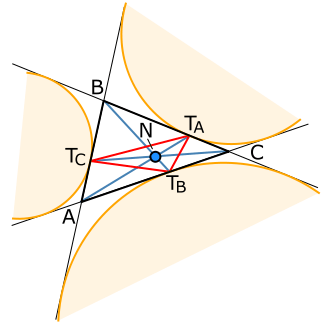# Splitter (geometry)The Nagel point (blue, N) of a triangle (black). The red triangle is the extouch triangle, and the orange circles are the excircles. The splitters of the perimeter are ATA, BTB, and CTC.

In plane geometry, a splitter is a line segment through one of the vertices of a triangle (that is, a cevian) that bisects the perimeter of the triangle.

## Properties

The opposite endpoint of a splitter to the chosen triangle vertex lies at the point on the triangle's side where one of the excircles of the triangle is tangent to that side. This point is also called a splitting point of the triangle. It is additionally a vertex of the extouch triangle and one of the points where the Mandart inellipse is tangent to the triangle side.

The three splitters concur at the Nagel point of the triangle, which is also called its splitting center.

## Generalization

Some authors have used the term "splitter" in a more general sense, for any line segment that bisects the perimeter of the triangle. Other line segments of this type include the cleavers, which are perimeter-bisecting segments that pass through the midpoint of a triangle side, and the equalizers, segments that bisect both the area and perimeter of a triangle.

## References

1. Honsberger, Ross (1995), "Chapter 1: Cleavers and Splitters", Episodes in Nineteenth and Twentieth Century Euclidean Geometry, Mathematical Association of America, pp. 1–14.
2. Avishalom, Dov (1963), "The perimetric bisection of triangles", Mathematics Magazine, 36 (1): 60–62, JSTOR 2688140, MR 1571272.
3. Juhász, Imre (2012), "Control point based representation of inellipses of triangles" (PDF), Annales Mathematicae et Informaticae, 40: 37–46, MR 3005114.
4. Kodokostas, Dimitrios (2010), "Triangle equalizers", Mathematics Magazine, 83 (2): 141–146, doi:10.4169/002557010X482916.